Home > CCA2 > Chapter 10 > Lesson 10.2.2 > Problem10-118

10-118.
1. Given seven points in a plane, no three of which are collinear: Homework Help ✎

1. How many different lines are determined by these points?

2. How many distinct triangles can be formed?

3. How many distinct quadrilaterals can be formed?

4. Explain why the answers to parts (b) and (c) are the same.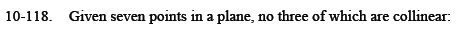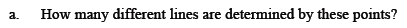'No three are colinear' means that any line drawn through two of the points will not intersect any of the other 5 points.

In order for a line to be determined by these points, it must pass through at least two of them.

Write a statement in the form of nCr to describe the situation.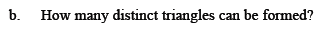Write a statement to describe choosing 3 out of the 7 points.

7C3 = 35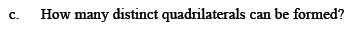Write a statement to describe choosing 4 out of the 7 points.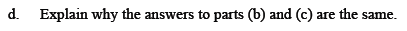Write the answers to parts (b) and (c) in nCr form, and then in factorial form.
Instead of simplifying, look at the similarities between the expressions.